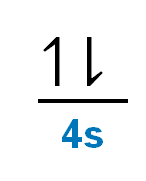# Problem: Which of the following is paramagnetic?CaCa2+Ti2+Zn2+Zn

###### FREE Expert Solution

To determine which is paramagnetic.

Recall that for:

• diamagnetic: all of the electrons are paired

• paramagnetic: at least one electron is unpaired

Note that for a neutral element, Atomic Number = # of protons = # of electrons.

For Ca:

Step 1: Electron configuration

The atomic number of Ca is 20, which means Ca has 20 e.

The noble gas before Ca is Ar (18 e)

The shorthand e config. for Ca is: Ca: [Ar]4s2

Step 2: Orbital diagram

The outermost sublevel is 4s. Recall that the s sublevel contains 1 orbital

When filling up the orbitals, take note of:

• Aufbau Principle: totally fill in the lowest energy orbital first

• Hund’s Rule: electron orbitals that are degenerate (same energy orbital) are first half-filled before they are totally filled

The orbital diagram for Ca is:100% (82 ratings)###### Problem Details

Which of the following is paramagnetic?

Ca

Ca2+

Ti2+

Zn2+

Zn